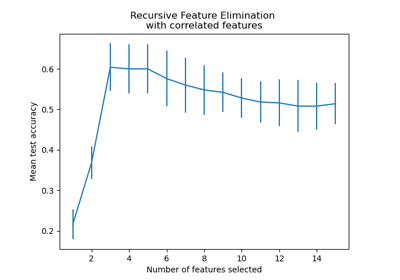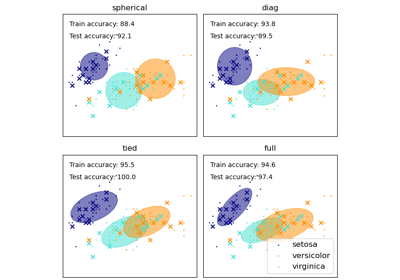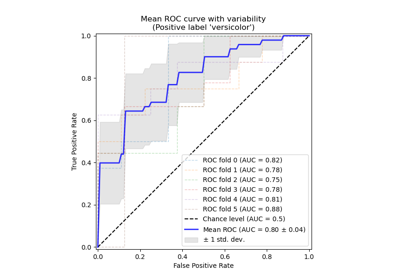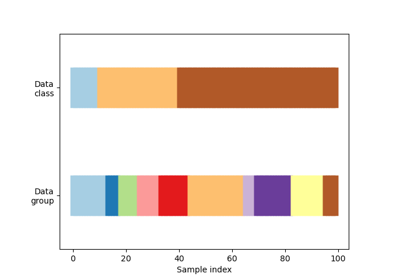# sklearn.model_selection.StratifiedKFold¶

class sklearn.model_selection.StratifiedKFold(n_splits=5, *, shuffle=False, random_state=None)[source]

Stratified K-Folds cross-validator

Provides train/test indices to split data in train/test sets.

This cross-validation object is a variation of KFold that returns stratified folds. The folds are made by preserving the percentage of samples for each class.

Read more in the User Guide.

Parameters
n_splitsint, default=5

Number of folds. Must be at least 2.

Changed in version 0.22: n_splits default value changed from 3 to 5.

shufflebool, default=False

Whether to shuffle each class’s samples before splitting into batches. Note that the samples within each split will not be shuffled.

random_stateint or RandomState instance, default=None

When shuffle is True, random_state affects the ordering of the indices, which controls the randomness of each fold for each class. Otherwise, leave random_state as None. Pass an int for reproducible output across multiple function calls. See Glossary.

RepeatedStratifiedKFold

Repeats Stratified K-Fold n times.

Notes

The implementation is designed to:

• Generate test sets such that all contain the same distribution of classes, or as close as possible.

• Be invariant to class label: relabelling y = ["Happy", "Sad"] to y = [1, 0] should not change the indices generated.

• Preserve order dependencies in the dataset ordering, when shuffle=False: all samples from class k in some test set were contiguous in y, or separated in y by samples from classes other than k.

• Generate test sets where the smallest and largest differ by at most one sample.

Changed in version 0.22: The previous implementation did not follow the last constraint.

Examples

>>> import numpy as np
>>> from sklearn.model_selection import StratifiedKFold
>>> X = np.array([[1, 2], [3, 4], [1, 2], [3, 4]])
>>> y = np.array([0, 0, 1, 1])
>>> skf = StratifiedKFold(n_splits=2)
>>> skf.get_n_splits(X, y)
2
>>> print(skf)
StratifiedKFold(n_splits=2, random_state=None, shuffle=False)
>>> for train_index, test_index in skf.split(X, y):
...     print("TRAIN:", train_index, "TEST:", test_index)
...     X_train, X_test = X[train_index], X[test_index]
...     y_train, y_test = y[train_index], y[test_index]
TRAIN: [1 3] TEST: [0 2]
TRAIN: [0 2] TEST: [1 3]


Methods

 get_n_splits([X, y, groups]) Returns the number of splitting iterations in the cross-validator split(X, y[, groups]) Generate indices to split data into training and test set.
__init__(n_splits=5, *, shuffle=False, random_state=None)[source]

Initialize self. See help(type(self)) for accurate signature.

get_n_splits(X=None, y=None, groups=None)[source]

Returns the number of splitting iterations in the cross-validator

Parameters
Xobject

Always ignored, exists for compatibility.

yobject

Always ignored, exists for compatibility.

groupsobject

Always ignored, exists for compatibility.

Returns
n_splitsint

Returns the number of splitting iterations in the cross-validator.

split(X, y, groups=None)[source]

Generate indices to split data into training and test set.

Parameters
Xarray-like of shape (n_samples, n_features)

Training data, where n_samples is the number of samples and n_features is the number of features.

Note that providing y is sufficient to generate the splits and hence np.zeros(n_samples) may be used as a placeholder for X instead of actual training data.

yarray-like of shape (n_samples,)

The target variable for supervised learning problems. Stratification is done based on the y labels.

groupsobject

Always ignored, exists for compatibility.

Yields
trainndarray

The training set indices for that split.

testndarray

The testing set indices for that split.

Notes

Randomized CV splitters may return different results for each call of split. You can make the results identical by setting random_state to an integer.

## Examples using sklearn.model_selection.StratifiedKFold¶